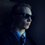# Place value function

This has probably already been done before, but I had lots of fun discovering it. :)

I wanted to create a function $f$, which, given a number $n$, would take in the place value of a digit $d$ of $n$ ($x$, such that the place value of $d$ is $10^{x}$), and output $d$ itself.

For example, if $n = 1345.829$, than $f(0) = 5$, $f(3) = 1$, and $f(-2) = 2$.

Here's what I came up with:

$f(x) = \dfrac{n \operatorname{mod} 10^{x+1} - n \operatorname{mod} 10^{x}}{10^{x}}$

And a Desmos graph.

I came up with it somewhat inductively, noting patterns while calculating the units digit, the tens digit, and so on, but I understand now why it works. The function essentially chops off everything after the desired place value, subtracts from that everything before the desired place value, and then divides by the power of ten associated with that place value. For example:

$n = 1345.829$, $x = 2$ (should retrieve the digit at $10^2$, or $3$)

$n \operatorname{mod} 10^{2+1} = 345.829$

$n \operatorname{mod} 10^{2} = 45.829$

$345.829 - 45.829 = 300.000$

$\frac{300.000}{10^{2}} = \boxed{3}$Note by David Stiff
1 month, 1 week ago

This discussion board is a place to discuss our Daily Challenges and the math and science related to those challenges. Explanations are more than just a solution — they should explain the steps and thinking strategies that you used to obtain the solution. Comments should further the discussion of math and science.

When posting on Brilliant:

• Use the emojis to react to an explanation, whether you're congratulating a job well done , or just really confused .
• Ask specific questions about the challenge or the steps in somebody's explanation. Well-posed questions can add a lot to the discussion, but posting "I don't understand!" doesn't help anyone.
• Try to contribute something new to the discussion, whether it is an extension, generalization or other idea related to the challenge.
• Stay on topic — we're all here to learn more about math and science, not to hear about your favorite get-rich-quick scheme or current world events.

MarkdownAppears as
*italics* or _italics_ italics
**bold** or __bold__ bold
- bulleted- list
• bulleted
• list
1. numbered2. list
1. numbered
2. list
Note: you must add a full line of space before and after lists for them to show up correctly
paragraph 1paragraph 2

paragraph 1

paragraph 2

[example link](https://brilliant.org)example link
> This is a quote
This is a quote
    # I indented these lines
# 4 spaces, and now they show
# up as a code block.

print "hello world"
# I indented these lines
# 4 spaces, and now they show
# up as a code block.

print "hello world"
MathAppears as
Remember to wrap math in $$ ... $$ or $ ... $ to ensure proper formatting.
2 \times 3 $2 \times 3$
2^{34} $2^{34}$
a_{i-1} $a_{i-1}$
\frac{2}{3} $\frac{2}{3}$
\sqrt{2} $\sqrt{2}$
\sum_{i=1}^3 $\sum_{i=1}^3$
\sin \theta $\sin \theta$
\boxed{123} $\boxed{123}$

## Comments

Sort by:

Top Newest

Now you can trick people by making it look a little more complicated using the method I had shown earlier using trig and inverse trig, making it non-obvious to the naked eye(the formula you have shown is what I normally use for getting digits in a computer program, but I was just reminded that I had found the modulus function in terms of trig and inverse trig)

- 1 month, 1 week ago

Log in to reply

How sinister! :)

I don't seem to remember seeing a note about that... could you add the link here?

- 1 month, 1 week ago

Log in to reply

It was actually in a daily challenge, in a comment as a reply to Siddarth’s comment(one or two fire), I don’t seem to remember the name so I have to go on treasure hunt too

- 1 month, 1 week ago

Log in to reply

Aha found it(joking it was quite recent) First solution, first comment, first reply to that, first reply to the first reply

- 1 month, 1 week ago

Log in to reply

Rats, I'm not a Premium member. It says the problem has expired. :/

- 1 month, 1 week ago

Log in to reply

Oh in that case I will put down here what I did there

- 1 month, 1 week ago

Log in to reply

"A better one is $\frac{10}{π}\cot^{-1}(\cot(\frac{3π}{10}x))$ "

"Got the perfect one $5+\frac{10}{π}(\tan^{-1}(\tan(\frac{3π}{10}x-\frac{π}{2})))$ "

These are both for $3x \mod {10}$, a little modification should get them to $x \mod {10^n}$

- 1 month, 1 week ago

Log in to reply

Very cool! Confusing at first too. :)

- 1 month, 1 week ago

Log in to reply

I tried out the graph using this function, it didn’t look good

- 1 month, 1 week ago

Log in to reply

Hm. For the trig one you mean?

- 1 month, 1 week ago

Log in to reply

Yeah I tried the trig, but I assume neither will look good

- 1 month, 1 week ago

Log in to reply

Yeah, the simpler one looks the same I think. It only really looks nice if you keep $n$ constant and then graph $f(x)$ for all integers between like $x = -10$ and $x = 10$ (or something else depending on $n$).

- 1 month, 1 week ago

Log in to reply

In fact when you add or subtract inverse trig stuff, it can be simplified to a single inverse trig expression, so after that it will look all the more confusing

- 1 month, 1 week ago

Log in to reply

×

Problem Loading...

Note Loading...

Set Loading...